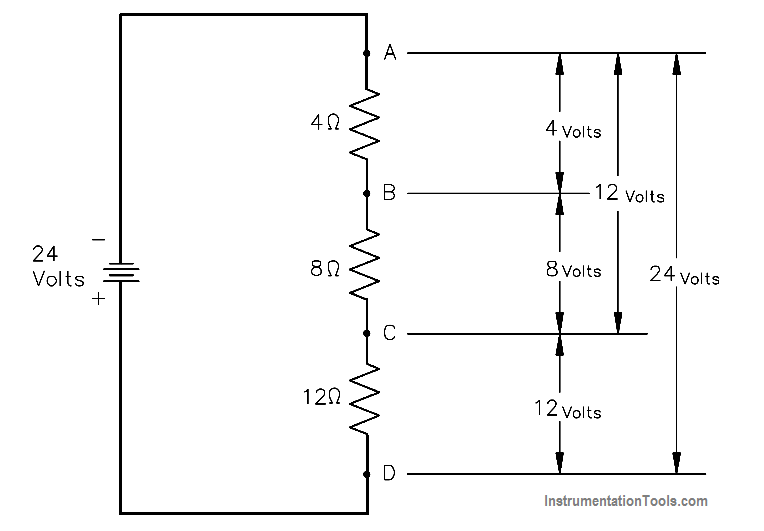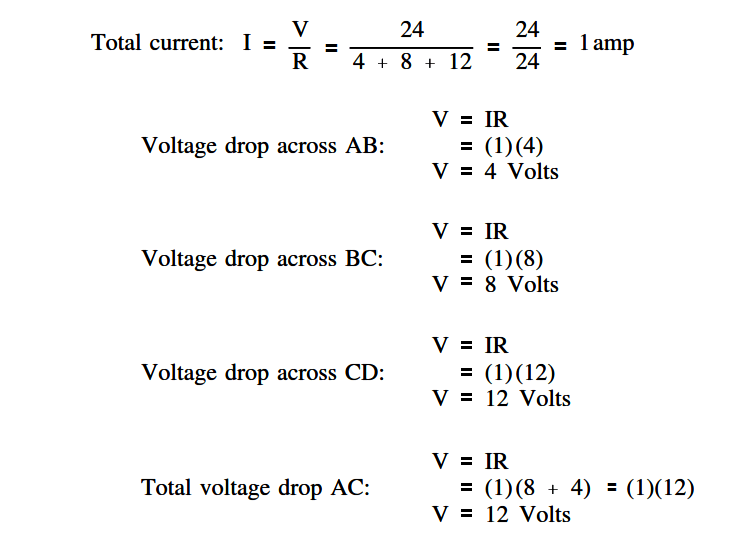# Voltage Divider

A voltage divider, or network, is used when it is necessary to obtain different values of voltage from a single energy source. A simple voltage divider is shown in Figure 29.

In this circuit, 24 volts is applied to three resistors in series. The total resistance limits the current through the circuit to one ampere. Individual voltages are found as follows using below equation.Figure 29 Voltage Divider